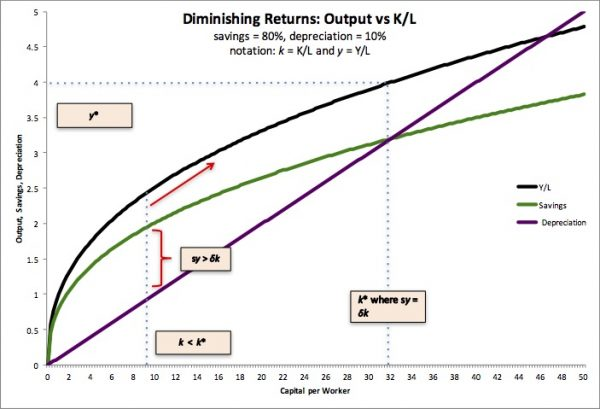# Solow Model

Published on

Here are the graphs and memo and the underlying spreadsheet.

1. the memo on Solow growth models where you can find details on the algebra.
2. The Solow Basic Excel spreadsheet.
3. Basic Production function showing diminishing returns and equilibrium output where new investment only just offsets depreciation.4. How fast does it converge? I took the basic framework and turned it into an excel spreadsheet. For this set:
• Yt-1 = At-1Kαt-1L1-αt-1.
• It-1=sYt-1.
• Kt=Kt-1-δKt-1+It-1
• With constant L and A you can then calculate the next row, and replicate to do the next 100…

The bottom line is that it converges quickly. Investment-led growth doesn’t last for long.5. If you let the LF grow (here at a constant percentage rate) the equilibrium level of output per person is a bit lower, but not by much. Otherwise the dynamics are identical.6. Put in productivity growth and everything changes. This growth has the LF expanding at 1% pa, as above, which pulls down growth. Add in even 0.5% pa productivity growth and suddenly you keep going past the 0.75 level at which output stabilized in our LF growth case. As per the rule of 70, at 0.5% growth it takes 140 years to double, but that’s close to 25% over a 40-year working life. Not good, but enough to be noticeable.